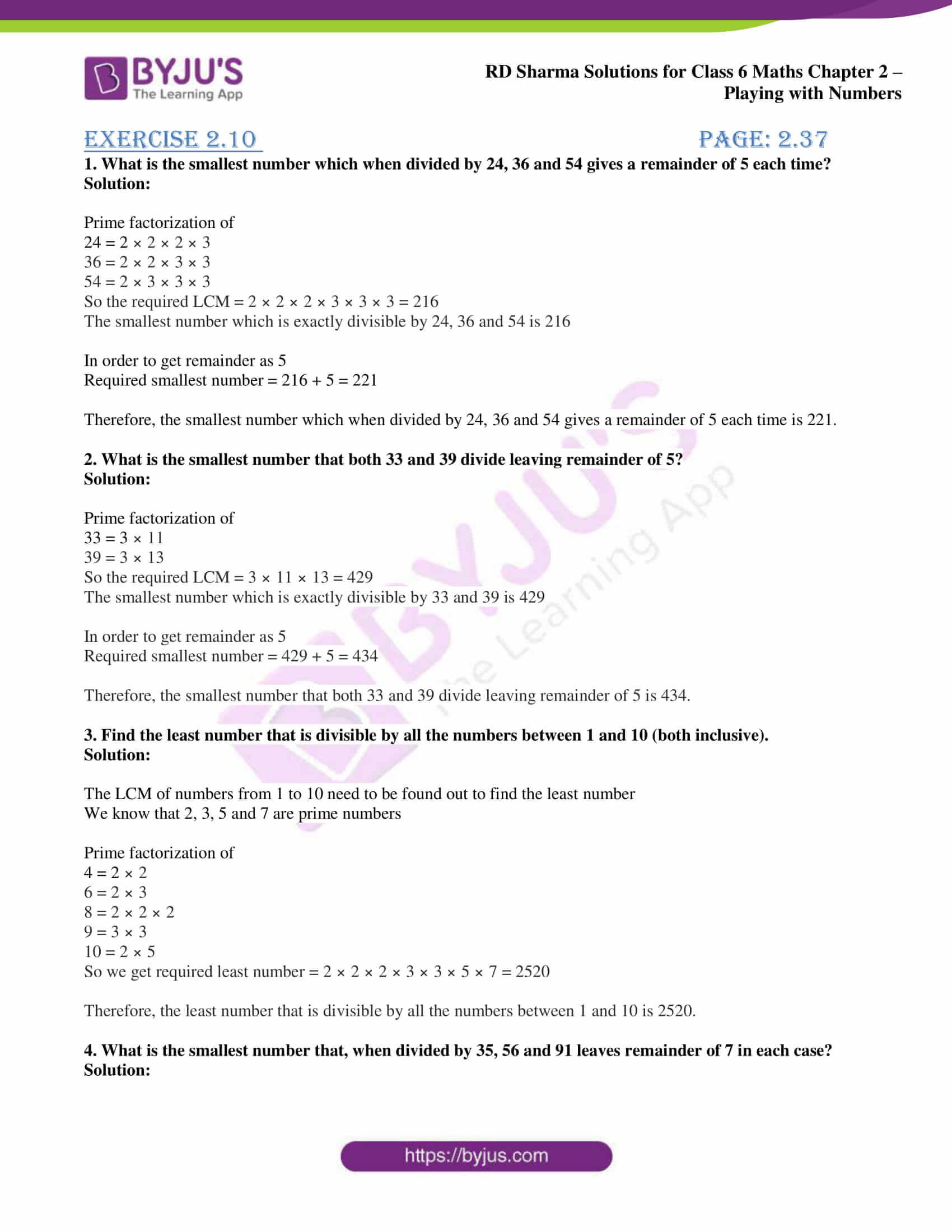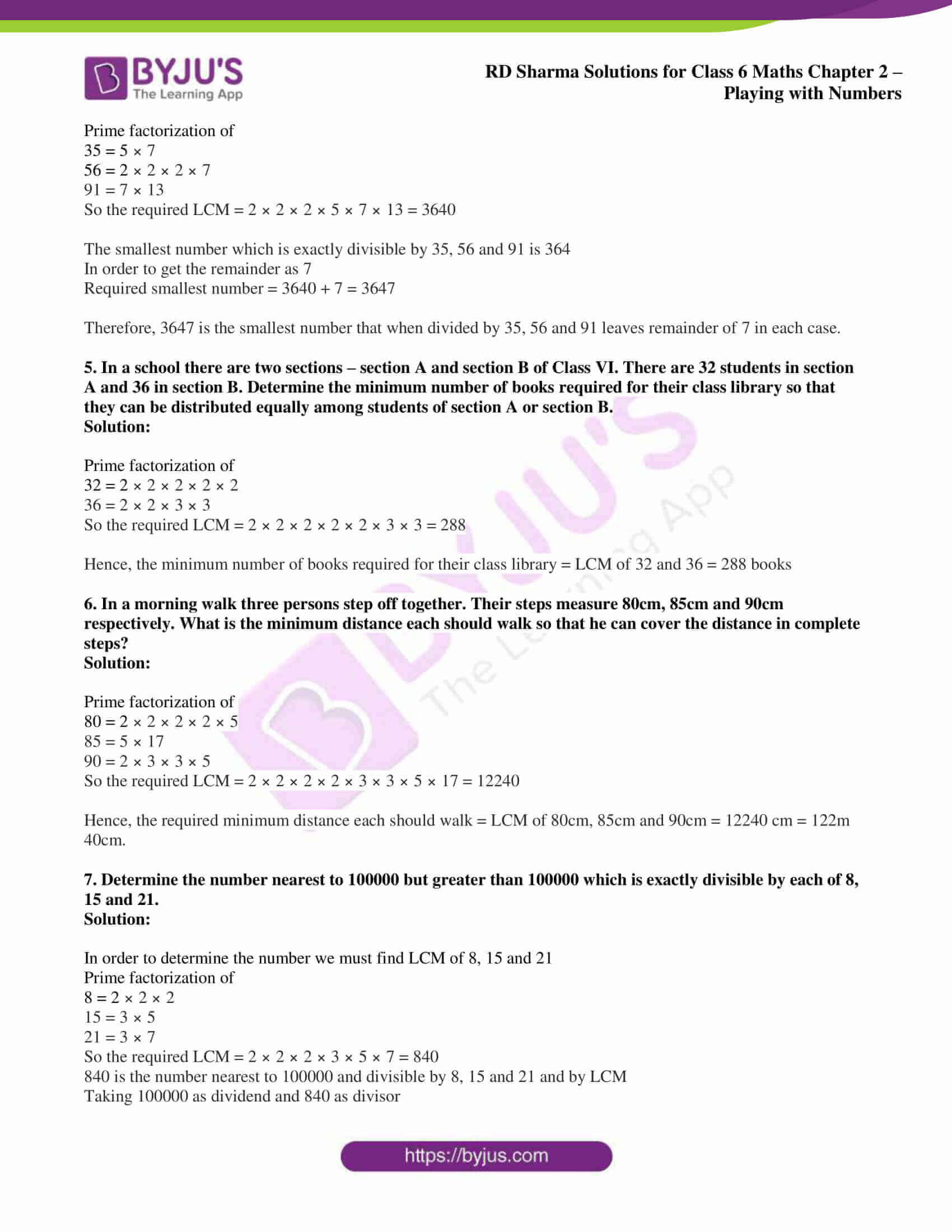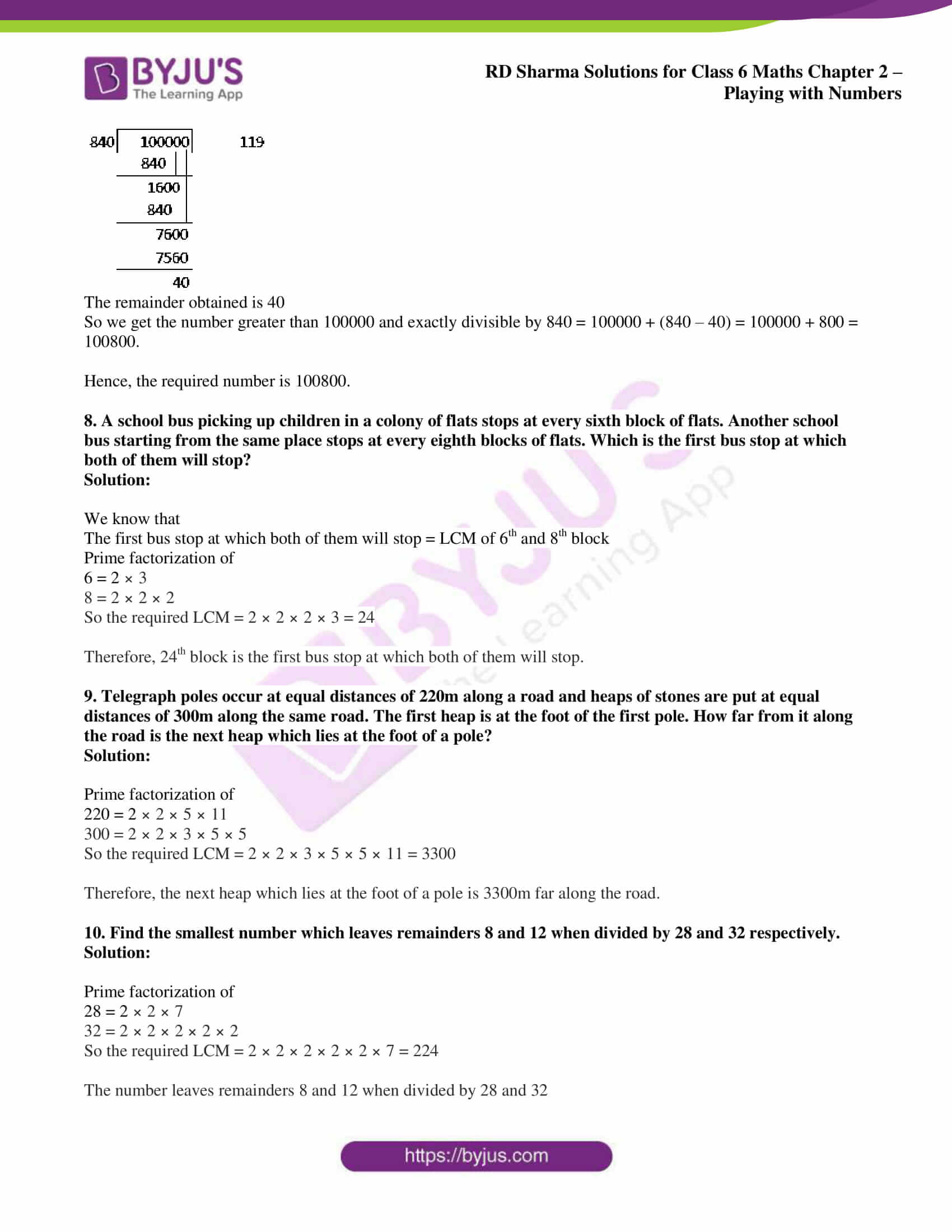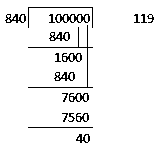# RD Sharma Solutions for Class 6 Maths Chapter 2: Playing with Numbers Exercise 2.10

## RD Sharma Solutions for Class 6 Maths Exercise 2.10 PDF

Mathematics is one among the important subjects which consists of various concepts that should be understood. The students are provided with exercise wise solutions which can be used in solving the problems of RD Sharma textbook. The solutions are in a step wise and explanatory manner to improve conceptual knowledge among students. The faculty at BYJU’S design the solutions with the aim of making the subject easier for the students to score good marks. RD Sharma Solutions for Class 6 Maths Chapter 2 Playing with Numbers Exercise 2.10 are provided here.

## RD Sharma Solutions for Class 6 Maths Chapter 2: Playing with Numbers Exercise 2.10 Download PDF## Access answers to Maths RD Sharma Solutions for Class 6 Chapter 2: Playing with Numbers Exercise 2.10

1. What is the smallest number which when divided by 24, 36 and 54 gives a remainder of 5 each time?

Solution:

Prime factorization of

24 = 2 × 2 × 2 × 3

36 = 2 × 2 × 3 × 3

54 = 2 × 3 × 3 × 3

So the required LCM = 2 × 2 × 2 × 3 × 3 × 3 = 216

The smallest number which is exactly divisible by 24, 36 and 54 is 216

In order to get remainder as 5

Required smallest number = 216 + 5 = 221

Therefore, the smallest number which when divided by 24, 36 and 54 gives a remainder of 5 each time is 221.

2. What is the smallest number that both 33 and 39 divide leaving remainder of 5?

Solution:

Prime factorization of

33 = 3 × 11

39 = 3 × 13

So the required LCM = 3 × 11 × 13 = 429

The smallest number which is exactly divisible by 33 and 39 is 429

In order to get remainder as 5

Required smallest number = 429 + 5 = 434

Therefore, the smallest number that both 33 and 39 divide leaving remainder of 5 is 434.

3. Find the least number that is divisible by all the numbers between 1 and 10 (both inclusive).

Solution:

The LCM of numbers from 1 to 10 need to be found out to find the least number

We know that 2, 3, 5 and 7 are prime numbers

Prime factorization of

4 = 2 × 2

6 = 2 × 3

8 = 2 × 2 × 2

9 = 3 × 3

10 = 2 × 5

So we get required least number = 2 × 2 × 2 × 3 × 3 × 5 × 7 = 2520

Therefore, the least number that is divisible by all the numbers between 1 and 10 is 2520.

4. What is the smallest number that, when divided by 35, 56 and 91 leaves remainder of 7 in each case?

Solution:

Prime factorization of

35 = 5 × 7

56 = 2 × 2 × 2 × 7

91 = 7 × 13

So the required LCM = 2 × 2 × 2 × 5 × 7 × 13 = 3640

The smallest number which is exactly divisible by 35, 56 and 91 is 364

In order to get the remainder as 7

Required smallest number = 3640 + 7 = 3647

Therefore, 3647 is the smallest number that when divided by 35, 56 and 91 leaves remainder of 7 in each case.

5. In a school there are two sections – section A and section B of Class VI. There are 32 students in section A and 36 in section B. Determine the minimum number of books required for their class library so that they can be distributed equally among students of section A or section B.

Solution:

Prime factorization of

32 = 2 × 2 × 2 × 2 × 2

36 = 2 × 2 × 3 × 3

So the required LCM = 2 × 2 × 2 × 2 × 2 × 3 × 3 = 288

Hence, the minimum number of books required for their class library = LCM of 32 and 36 = 288 books

6. In a morning walk three persons step off together. Their steps measure 80cm, 85cm and 90cm respectively. What is the minimum distance each should walk so that he can cover the distance in complete steps?

Solution:

Prime factorization of

80 = 2 × 2 × 2 × 2 × 5

85 = 5 × 17

90 = 2 × 3 × 3 × 5

So the required LCM = 2 × 2 × 2 × 2 × 3 × 3 × 5 × 17 = 12240

Hence, the required minimum distance each should walk = LCM of 80cm, 85cm and 90cm = 12240 cm = 122m 40cm.

7. Determine the number nearest to 100000 but greater than 100000 which is exactly divisible by each of 8, 15 and 21.

Solution:

In order to determine the number we must find LCM of 8, 15 and 21

Prime factorization of

8 = 2 × 2 × 2

15 = 3 × 5

21 = 3 × 7

So the required LCM = 2 × 2 × 2 × 3 × 5 × 7 = 840

840 is the number nearest to 100000 and divisible by 8, 15 and 21 and by LCM

Taking 100000 as dividend and 840 as divisorThe remainder obtained is 40

So we get the number greater than 100000 and exactly divisible by 840 = 100000 + (840 – 40) = 100000 + 800 = 100800.

Hence, the required number is 100800.

8. A school bus picking up children in a colony of flats stops at every sixth block of flats. Another school bus starting from the same place stops at every eighth blocks of flats. Which is the first bus stop at which both of them will stop?

Solution:

We know that

The first bus stop at which both of them will stop = LCM of 6th and 8th block

Prime factorization of

6 = 2 × 3

8 = 2 × 2 × 2

So the required LCM = 2 × 2 × 2 × 3 = 24

Therefore, 24th block is the first bus stop at which both of them will stop.

9. Telegraph poles occur at equal distances of 220m along a road and heaps of stones are put at equal distances of 300m along the same road. The first heap is at the foot of the first pole. How far from it along the road is the next heap which lies at the foot of a pole?

Solution:

Prime factorization of

220 = 2 × 2 × 5 × 11

300 = 2 × 2 × 3 × 5 × 5

So the required LCM = 2 × 2 × 3 × 5 × 5 × 11 = 3300

Therefore, the next heap which lies at the foot of a pole is 3300m far along the road.

10. Find the smallest number which leaves remainders 8 and 12 when divided by 28 and 32 respectively.

Solution:

Prime factorization of

28 = 2 × 2 × 7

32 = 2 × 2 × 2 × 2 × 2

So the required LCM = 2 × 2 × 2 × 2 × 2 × 7 = 224

The number leaves remainders 8 and 12 when divided by 28 and 32

So we get

28 – 8 = 20 and 32 – 12 = 20

Hence, the required number is 224 – 20 = 204

### RD Sharma Solutions for Class 6 Maths Chapter 2 – Playing with Numbers Exercise 2.10

RD Sharma Solutions Class 6 Maths Chapter 2 Playing with Numbers Exercise 2.10 helps students to understand the major applications of LCM in daily life.

### Key features of RD Sharma Solutions for Class 6 Maths Chapter 2: Playing with Numbers Exercise 2.10

• It helps students understand the concepts which are covered under each exercise and perform well in the exam.
• The subject experts at BYJU’S create solutions which are accurate based on the latest syllabus of CBSE board.
• Practising lots of problems help students remember the methods which are used in solving problems.
• Conceptual knowledge and problem solving abilities are improved among students, which significantly contributes to scoring good marks.

1. Arnav Singh

The length, breadth and width of a room are 80 m, 85 m and 90 m respectively. Find the length of the largest tape that can measure the three dimensions of the room exactly.

• Length = 80 cm = 2 × 2 × 2 × 2 × 5

Breadth = 85 cm = 5 × 17

Height = 90 cm = 2 × 3 × 3 × 5

Longest tape = HCF of 80, 85, and 90 = 5 cm

Therefore, the longest tape is 5 cm.